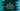# C# program to find the average of odd and even numbers in an array## How to find the average of odd and even numbers in an array in C#:

In this post, we will learn how to find the average of all odd and even numbers in a C# array. Our program will take the numbers as input from the user, insert them in the array and find out the average of all odd values and even values.

To solve this problem, we can use a loop to iterate over the numbers and find the sum and count for both even and odd. Once we calculate the sum and count for both, we can find the average by dividing sum by count.

This problem can be solved by using any loop. In this post, I will show you how to use a for loop or foreach loop to do that.

### C# program to find the average of odd and even numbers in an array:

Let’s take a look at the below program:

``````using System;
public class Program {
public static void Main() {
int size;
int oddSum = 0, evenSum = 0;
int oddCount = 0, evenCount = 0;

Console.WriteLine("Enter the size of the array:");

int[] arr = new int[size];

Console.WriteLine("Enter the array values:");
for(int i = 0; i< size; i++){
}

for(int i = 0; i< size; i++){
if(arr[i] % 2 == 0){
evenSum += arr[i];
evenCount++;
}else{
oddSum += arr[i];
oddCount++;
}
}

Console.WriteLine("Even average: "+evenSum/evenCount+" Odd average: "+oddSum/oddCount);
}
}``````

Here,

• size is an integer value to store the size of the array.
• oddSum and evenSum are to hold the sum of the odd and even values in the array. oddCount and evenCount are to hold the count of odd and even values in the array.
• It is asking the user to enter the size of the array and reading it to the size variable.
• arr is an integer array of size equal to what the user entered in the last step. We will store all inputs in this array.
• Using a for loop, we are reading the numbers entered by the user and storing it in arr.
• The second for loop is to scan the array elements one more time after the user entered all values. For each element, we are checking if it is even or not. If it is even, we are adding that value to evenSum and incrementing the value of evenCount by 1. Similarly, if it is odd, we are adding it to oddSum and incrementing the value of oddCount by 1.
• The final WriteLine is printing the average of all even numbers and odd numbers.

If you run this program, it will print the output as like below:

``````Enter the size of the array:
7
Enter the array values:
1
2
3
4
5
6
7
Even average: 4 Odd average: 4``````

### By using a foreach loop:

We can also use a foreach loop instead of a for loop. Below is the complete program:

``````using System;
public class Program {
public static void Main() {
int size;
int oddSum = 0, evenSum = 0;
int oddCount = 0, evenCount = 0;

Console.WriteLine("Enter the size of the array:");

int[] arr = new int[size];

Console.WriteLine("Enter the array values:");
for(int i = 0; i< size; i++){
}

foreach(int value in arr){
if(value % 2 == 0){
evenSum += value;
evenCount++;
}else{
oddSum += value;
oddCount++;
}
}

Console.WriteLine("Even average: "+evenSum/evenCount+" Odd average: "+oddSum/oddCount);
}
}``````

Here, we are using foreach to iterate through the items in the array. It is similar to the above program. The only difference is that we don’t have to use the index to get the value if we use foreach. Other things are same as the previous example.

You will get similar output with this approach.